# How To Read And Understand Electrical Schematics

Electrical schematics are essential for engineers and technicians to analyze, troubleshoot, and repair electrical circuits. Understanding electrical schematics is also important for anyone who is interested in understanding the technology behind many of the everyday items we use. Exploring the electrical schematic can provide insights into how the circuit works and how it could be improved. This article will explain the basics of reading electrical schematics, so that you can become familiar with the terminology and understand how the components of a circuit are connected.

## Basic Symbols Used in Electrical Schematics

The first step in learning to read electrical schematics is to become familiar with the symbols used on them. The symbols represent the components that make up the circuit and the wires that connect them together. Some of the most common symbols include: resistors, capacitors, transistors, and diodes. These symbols are usually represented by a graphic and a label. It is important to note that each symbol can have different meanings depending on the type of electrical circuit it represents.

## Layout of Electrical Schematics

Once you understand the basic symbols used in electrical schematics, the next step is to look for the layout of the circuit. Most schematics will have a layout or map showing the components and how they are connected together. This helps the reader to understand how the circuit is constructed and how it will work. For example, a resistor may be placed between two transistors, and a capacitor may be connected across two resistors. The layout of the circuit can help to identify any problems, such as a short circuit or an open circuit.

## Wiring Diagrams

In addition to the schematic, many circuits also require a wiring diagram. This is a schematic that shows the physical connection of the wires of the circuit. Wiring diagrams are useful for troubleshooting and repairing circuits because they allow the user to see exactly how the wires are connected. They also help to identify any loose connections or damaged wires that may be causing a problem.

## Reading and Interpreting Electrical Schematics

Once you understand the basic symbols used to represent components and the layout of the circuit, the next step is to read and interpret the electrical schematic. This can be done by looking for patterns and trends in the circuit. For example, if there is a series of connected resistors, then it is likely that the circuit is designed to regulate current or voltage. Similarly, if there is a group of transistors connected together, then it is likely that the circuit is designed to amplify a signal. By looking for these patterns, it is possible to gain a better understanding of how the circuit works.

## Troubleshooting Electrical Schematics

Troubleshooting electrical schematics involves understanding the components and how they are connected. Once the circuit is understood, then it is possible to identify any problems. This may involve testing the components with a multi-meter or replacing defective components. In some cases, it may be necessary to completely rewire the circuit to identify the cause of a malfunction.

## Conclusion

By understanding the basics of electrical schematics and being familiar with the symbols and layout of a circuit, it is possible to read and interpret electrical schematics. This knowledge can be used to troubleshoot circuits and identify any problems. As with any skill, the more practice and experience you get, the easier it will become.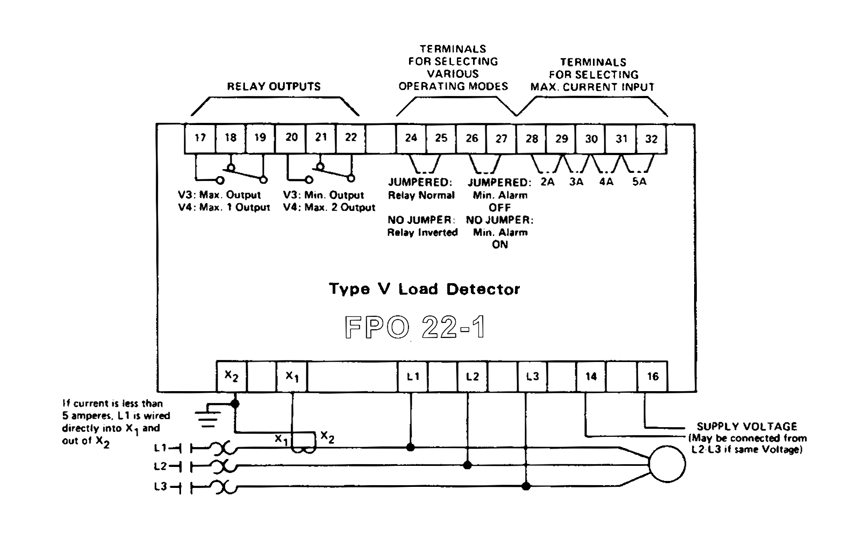Electrical Drawings And Schematics OverviewHow To Read A Schematic Learn Sparkfun Com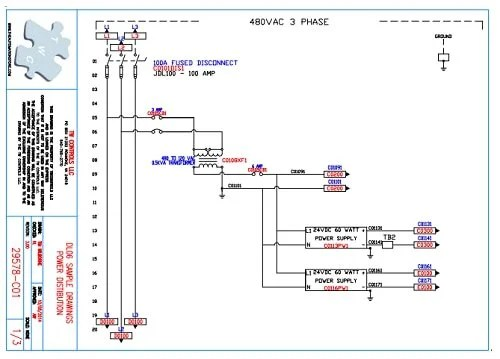Plc Training Reading Electrical Wiring Diagrams And Understanding Schematic Symbols Tw ControlsHow To Read A Schematic Learn Sparkfun ComHow To Read A Schematic Learn Sparkfun ComHow To Read Electrical Schematics Circuit BasicsInterpreting Electrical Schematics PetroedHow To Read Electrical Schematics Circuit BasicsThe Schematic Diagram A Basic Element Of Circuit Design Analog DevicesHow To Read A Schematic Learn Sparkfun Com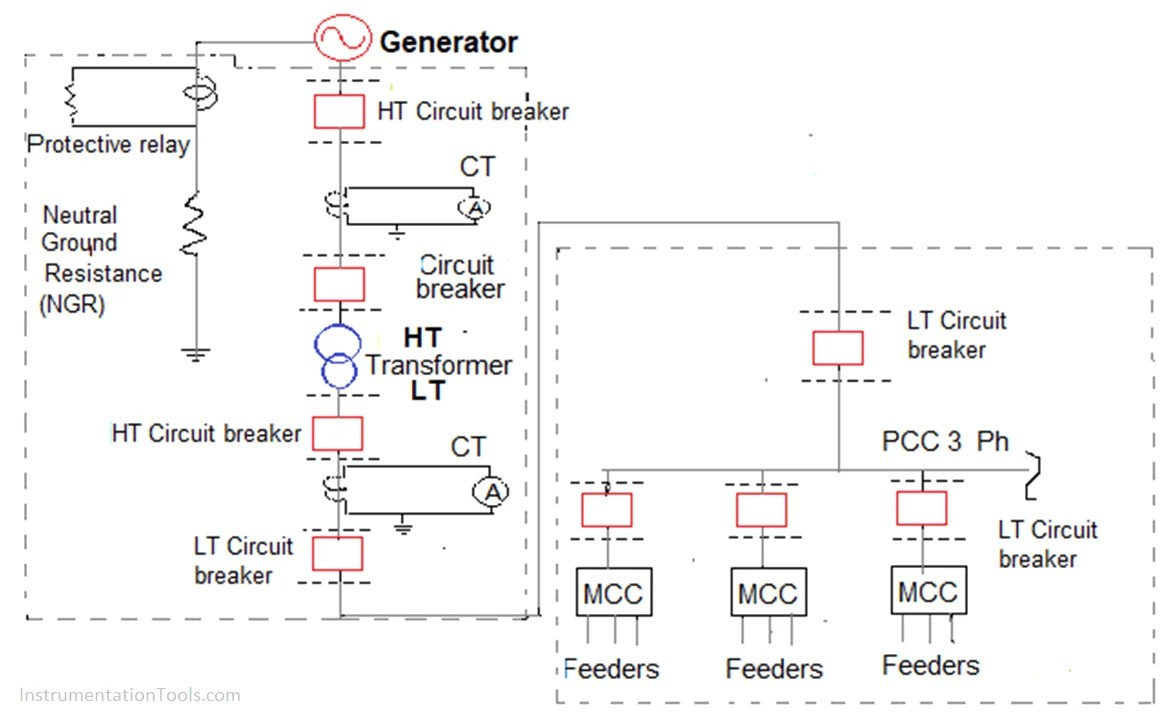How To Read And Understand An Electrical Single Line Diagram4 Ways To Read Schematics Wikihow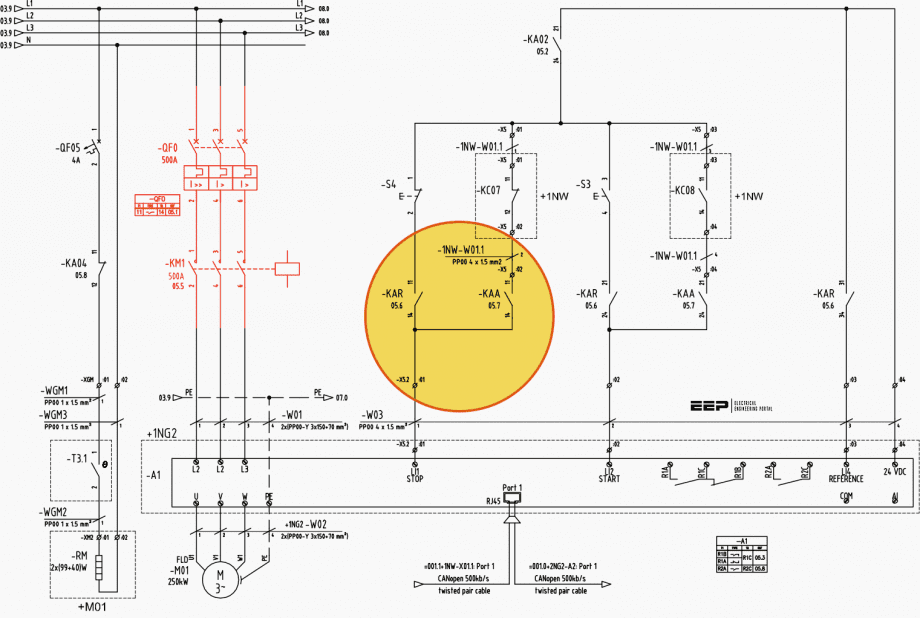Learn To Read And Understand Single Line Diagrams Wiring EepHow To Read Electrical Schematics Circuit BasicsHow To Read Electrical Schematics Circuit BasicsLearn To Interpret Single Line Diagram Sld Eep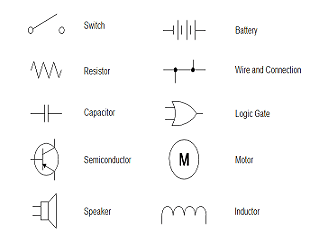How To Read And Understand Schematics In Electrical Basic Symbols Expressions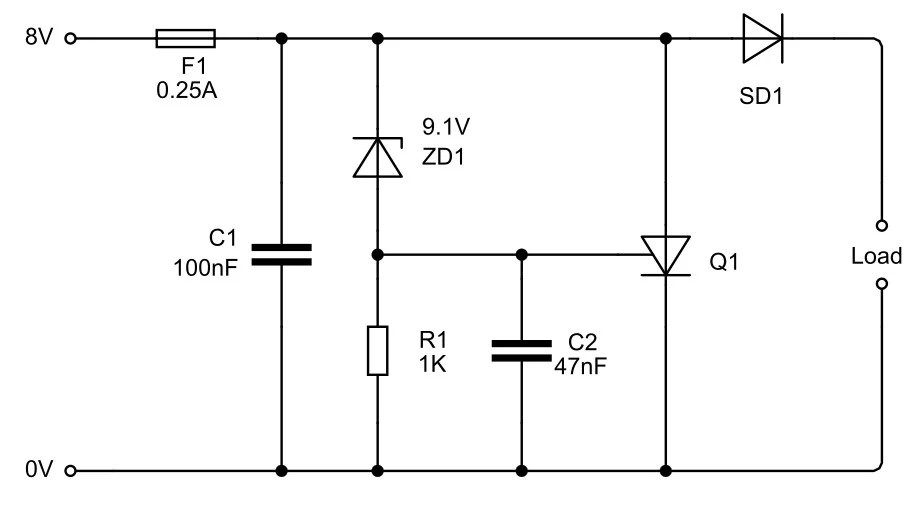July 2019 Elexhere4 Ways To Read Schematics Wikihow